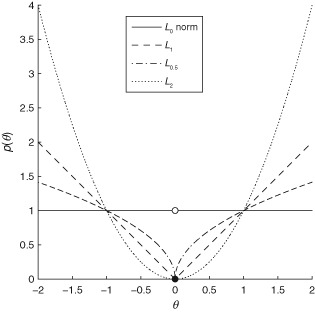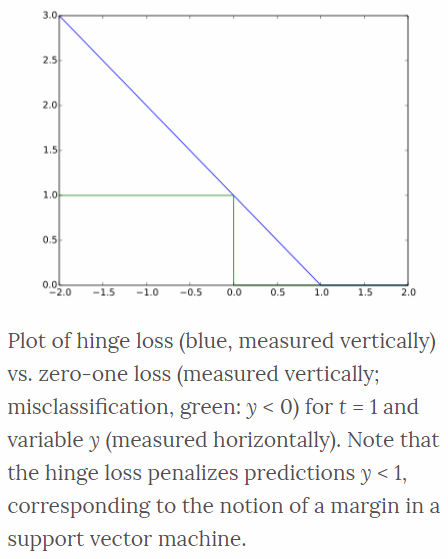When we train a deep learning model, we need to set a loss function for minimizing the error. The loss function indicates how much each variable contributes to the value to be optimized in the problem.

# Loss function

In deep learning context, the Loss function is a function that measures the quality of a particular set of parameters based on how well the output of the network agrees with the ground truth labels in the training data.

• The loss function is used to guide the training process in order to find a set of parameters that reduce the value of the loss function
• loss function = cost function = objective function = error function
• Loss function can be written as an average over loss functions for individual training examples:

## Empirical Risk Minimization (ERM)

Let a loss function $C(y, y')$ be given that penalizes deviations between the true class and the estimated one. The Empirical Risk (the average loss or error of an estimator) of a decision strategy is the total loss:

It should be minimized with respect to the decision strategy $e$.

# For Regression

For regression problem, network predicts continuous, and numeric variables. Loss functions for regression problem includes absolute value, square error, etc.

## L1-Norm (Absolute Value)

L1-norm loss function minimizes the sum of the absolute differences $\mathcal{L}$ between the target value $y_i$ and the estimated values $f_{\theta}(x_i)$.

• Pros
• Produces sparser solutions
• Good in high dimensional spaces
• Prediction speed
• Robust: less sensitive to outliers
• Cons
• Unstable solution (possibly multiple solutions)
• Computational inefficient on non-sparse cases

## L2-Norm (Square Error, Euclidean Loss)

L2-norm loss function minimize the sum of the square of the differences $\mathcal{L}$ between the target value $y_i$ and the estimated values $f_{\theta}(x_i)$.

• Pros
• More precise and better than L1-norm
• Penalizes large errors more strongly
• Stable solution (always one solution)
• Cons
• Sensitive to outliers
• Computational efficient due to having analytical solutions# For Classification

For classification problem, network predicts categorical variables. Loss function for classification problem includes hinges loss, cross-entropy loss, etc.

## Square Loss

Square loss is more commonly used in regression, but it can be utilized for classification by re-writing as a function $\phi(yf(\vec{x}))$.

The square loss function is both convex and smooth and matches the 0–1 when $yf(\vec{x})= 0$ and when $yf(\vec{x}) = 1$.

## Hinge Loss

The hinge loss is used for maximum-margin classification task, most notably for support vector machines (SVMs). For an intended output $t=±1$, a classifier score $y$ which should be the raw output of the classifier’s decision function, not the predicted class label.

For example, in linear SVMs, $y=wx+b$ where $(w,b)$ are the parameters of the hyperplane and $x$ is the point to classify. When $t$ and $y$ have the same sign ($y$ predicts the right class) and $\mid y \mid \geq 1$, the hinge loss $ell(y) = 0$. When they have opposite sign, $ell(y)$ increases linearly with $y$.## Logistic Loss

Logistic loss displays a similar convergence rate to the hinge loss function, and since it is continuous, gradient descent methods can be utilized. The logistic loss function is defined as

## Cross Entropy Loss

Cross entropy loss is commonly used loss function for deep neural network training. It is closely related to the Kullback-Leibler divergence between the empirical distribution and the predicted distribution. It is not naturally represented as a product of the true label and the predicted value, but it is convex and can be minimized using stochastic gradient descent methods. Using the alternative label convention $t=(1+y)/2$ so that $t \in {0,1}$, the cross entropy loss is defined as,

• Slide: Transformer: Loss functions (DLAI D4L2 2017 UPC Deep Learning for Artificial Intelligence) [Link]